Next: Electromagnetic Coupling Up: Interaction with the Electromagnetic Previous: Interaction with the Electromagnetic

## Gauge Invariance

Gauge invariance of Maxwell's equations in the quantum form of the theory is directly related to an invariance under a local phase transformation of the quantum fields. A generalized form of this phase invariance also underlies the theories of the weak and strong interactions. For this reason they are all known as gauge theories''.

In a global invariance the same transformation is carried out at all space-time points: it has an everywhere simultaneously'' character. In a local invariance, different transformations are carried out at different individual space-time points. For example, electric charge must be locally conserved. A process in which charge is created at one point and destroyed at a distant one is not allowed despite the fact that it conserves the charge overall or globally. The ultimate reason for this is that the global form of charge conservation would necessitate the instantaneous propagation of signals and this conflicts with special relativity.

The origin of gauge invariance in classical electromagnetism lies in the fact that the potentials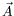and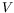are not unique for given physical fields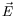and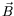. The transformations thatandmay undergo, while preservingandand hence Maxwell's equations, are called gauge transformations.

The Klein-Gordon equation is invariant under a phase transformation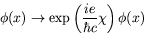(4.60)

provided that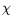is independent of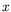. Ifis a function of, a derivative term gives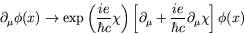(4.61)

and thus the Klein-Gordon equation is no longer invariant. Invariance may be restored by introducing a classical electromagnetic field,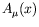, which transforms as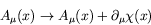(4.62)

under a gauge transformation. This is allowed in the standard Maxwell equations.

Using the so called minimal coupling'', the modified Klein-Gordon equation is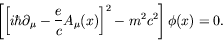(4.63)

The presence of the vector field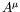, interacting in a universal'' prescribed way with any particle of charge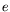, is dictated by local phase invariance. A vector field such as, introduced to guarantee local phase invariance, is called a gauge field''. The principle that the interaction should be so dictated by the phase (or group) invariance is called the gauge principle: it allows us to write down the wave equation for the interaction directly from the free particle equation via the replacement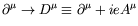, where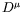is call the gauge covariant derivative'' usually just call the covariant derivative''.

The gauge transformation (4.62) produces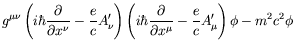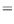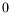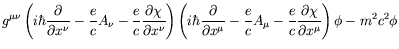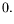(4.64)

Multiplying on the left-hand side of the equation with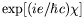and then rearranging so we are operating on the produced of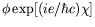, we have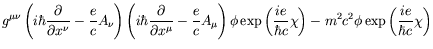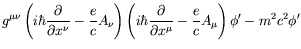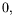(4.65)

where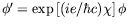. In other words the gauge transformation changes the phase of the wave function only. The phase factor is the same for all states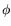, but not necessarily the same for all space-time points.

As we will show later, all physical observables are represented by bilinear forms with the structure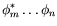and thus a common phase factor does not play any role in the physics. Thus we say that the Klein-Gordon equation is gauge invariant for the minimal coupling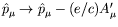.

General gauge invariance can be shown by first applying the gauge transformation once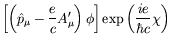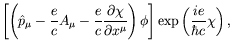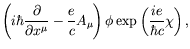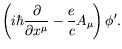(4.66)

For arbitrary powers of the operator,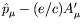,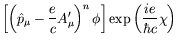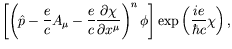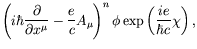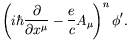(4.67)

Gauge invariance consequently also holds for arbitrary operator functions,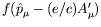, which can be expanded in a power series of theoperator. We can write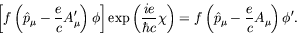(4.68)

Thus, minimal coupling is gauge invariant in a very general sense.Next: Electromagnetic Coupling Up: Interaction with the Electromagnetic Previous: Interaction with the Electromagnetic
Douglas M. Gingrich (gingrich@ ualberta.ca)
2004-03-18